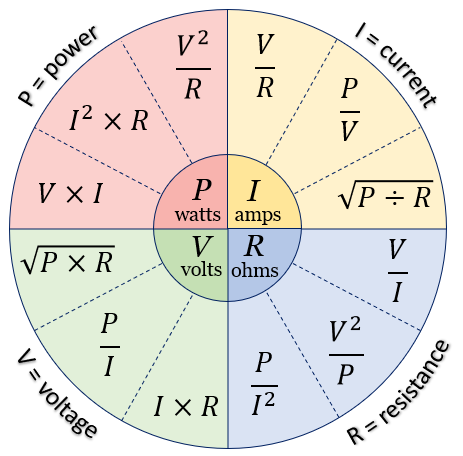# # Ohm's law calculator

This is a simple tool that allows you to calculate the voltage, current, resistance and power using Ohm's law.

Simply select and enter a vaule for 2 of the input fields.

x
x
x
x

...and here's an overview of the formulas used: